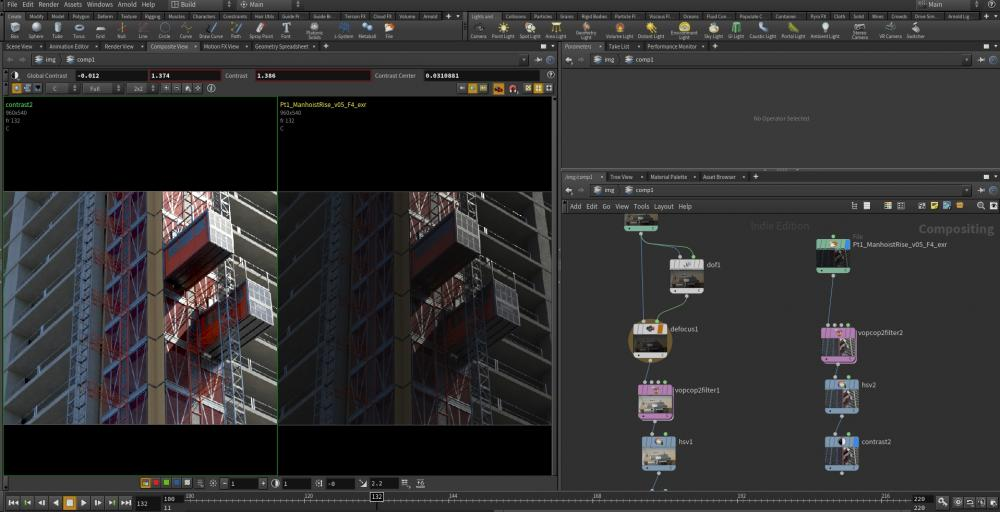## Recommended Posts

VEX code:

```
// Hable (Uncharted 2) Tonemapping
//
// Adapted from code by John Hable
// http://filmicgames.com/archives/75

vector hableTonemap(vector x)
{
float hA = 0.15;
float hB = 0.50;
float hC = 0.10;
float hD = 0.20;
float hE = 0.02;
float hF = 0.30;

return ((x*(hA*x+hC*hB)+hD*hE) / (x*(hA*x+hB)+hD*hF)) - hE/hF;
}

vector inputColor = set(_R, _G, _;

vector tonemapped = hableTonemap(_exposure * inputColor);

float hW = 11.2;
vector whiteScale = 1.0f / hableTonemap(hW);
tonemapped = tonemapped * whiteScale;

assign(_R, _G, _B, tonemapped);

```
```
// ACES Filmic Tone Mapping Curve
//
// Adapted from code by Krzysztof Narkowicz
// https://knarkowicz.wordpress.com/2016/01/06/
// aces-filmic-tone-mapping-curve/

vector ACESFilm( vector x )
{
float tA = 2.51f;
float tB = 0.03f;
float tC = 2.43f;
float tD = 0.59f;
float tE = 0.14f;
return clamp((x*(tA*x+tB))/(x*(tC*x+tD)+tE),0.0,1.0);
}

vector tonemapped = ACESFilm(set(_R,_G,_ * _exposure);
assign(_R, _G, _B, tonemapped);

```
•2

##### Share on other sites

Please tell me if the file works for you and if you have experience with other algorithms for tonemapping in Houdini.

Hey! Good stuff!

I did also some other ones at http://forums.odforce.net/topic/8471-eetus-lab/page-14#entry125594if you want to check 'em out..

##### Share on other sites

Whoa, greatSomehow I didn't come across your post when I searched for 'houdini tonemapping'. Having found nothing, I decided to write such a node.

##### Share on other sites

I edited the original post with an interesting ACES tonemapping. The pictures compare both algorithms with the original photo.

Here are also 1D LUT files (of 1024 length) that perform ACES curve. So you can use it straight in render viewRange is 0.001 - 10.0. Still untested but seems to be working.

(If used in a Lookup node, it requires 'Quantize' parameter to be set to 'Quantize at this node'. I don't know why.)

ACES_LUTs.zip

•1

##### Share on other sites

Hello Oskar, thank you very much for such an interesting thread, may I ask you, which tonemapper do you prefer? And how did you generate these LUTs? Thank you very much##### Share on other sites

Hi! I prefer ACES for personal projects or early (concept) renders - and Hable or S-Log for previewing work that will be sent further to compositing.

I'd like to build some custom tonemappers at some point. Like in "Advanced Techniques and Optimization of HDR Color Pipelines" by Timothy Lottes:

I generate LUTs for Houdini with a standalone Python script:

```# User options:
RANGE_MIN = 0.001   # Minimum input pixel brightness ()
RANGE_MAX = 10.0    # Max input pixel brightness value
LENGTH = 1024   # Number of LUT entries (precision)

EXPOSURE = 1.0  # Multiplier. Useful range is 0.125 - 8.0. Default is 1.0
OUTPUT_IN_SRGB = False # False means linear
TONEMAPPING = 'SLog'   # 'ACES', 'Hable' or 'SLog'

import math

def saturate(value):
return max(0.0, min(value, 1.0))
pass

def s_log_tonemap(x):
# Adapted from "S-Log: A new LUT for digital
# production mastering and interchange applications"
# https://pro.sony.com/bbsccms/assets/files/mkt/cinema/solutions/slog_manual.pdf
result = ((0.432699 * math.log10(x + 0.037584) + 0.616596) + 0.03)
return saturate(result)

def hable_tonemap_core(x):
# Hable (Uncharted 2) Tonemapping
# Adapted from code by John Hable
# http://filmicgames.com/archives/75
hA = 0.15
hB = 0.50
hC = 0.10
hD = 0.20
hE = 0.02
hF = 0.30
return ((x*(hA*x+hC*hB)+hD*hE) / (x*(hA*x+hB)+hD*hF)) - hE/hF

def hable_tonemap(x):
tonemapped = hable_tonemap_core(x)
hW = 11.2
whiteScale = 1.0 / hable_tonemap_core(hW)
return saturate(tonemapped * whiteScale)

def aces_tonemap(x):
# ACES Filmic Tone Mapping Curve
# Adapted from code by Krzysztof Narkowicz
# https://knarkowicz.wordpress.com/2016/01/06/
# aces-filmic-tone-mapping-curve/
tA = 2.51
tB = 0.03
tC = 2.43
tD = 0.59
tE = 0.14
result = (x * (tA*x + tB)) / (x * (tC*x + tD) + tE)
return saturate(result)

def linear_to_gamma_space(x):
return pow(x, 1/2.2)
pass

def gamma_to_linear_space(x):
return pow(x, 2.2)
pass

def encode_linear_to_log(idx):
# From Houdini HDK docs, "The Houdini LUT Format":
# p = e^(idx * (ln(in_end) - ln(in_start)) / (length-1) + log(in_start))
return pow(math.e, ( idx*( math.log(RANGE_MAX) - math.log(RANGE_MIN) ) / (LENGTH-1) + math.log(RANGE_MIN) ))

def calculate_values():
values = []

for idx in range(0, LENGTH):
exposureCorrected = EXPOSURE * encode_linear_to_log(idx)

result = 0.0
if TONEMAPPING == 'ACES':
result = aces_tonemap(exposureCorrected)
if OUTPUT_IN_SRGB:
result = linear_to_gamma_space(result)
elif TONEMAPPING == 'Hable':
result = hable_tonemap(exposureCorrected)
if OUTPUT_IN_SRGB:
result = linear_to_gamma_space(result)
elif TONEMAPPING == 'SLog':
result = s_log_tonemap(exposureCorrected)
if not OUTPUT_IN_SRGB:
result = gamma_to_linear_space(result)

values.append(result)

return values

def generate_text(values = []):
txt = [
"Version\t\t3",
"Format\t\tany",
"Type\t\tC",
"From\t\t" + str(RANGE_MIN) + " " + str(RANGE_MAX),
"To\t\t\t0 1",
"Black\t\t0",
"White\t\t1",
"Length\t\t" + str(LENGTH),
"Sampling\tLog",
"LUT:",
"RGB {"
]

for val in values:
txt.append("\t" + str(val))

txt[-1] += " }\n"

return ("\n").join(txt)```

•2

##### Share on other sites

I gave this a whirl in Houdini 16, and it works like a charm. The results of these tonemappers, which I rolled into a single VopCop node so that I can flip between them, are comparable to what I get out of Nuke. Thanks for posting these Oskar! You're a life saver. =]Edited by CrazyDraisey
Added second image showing the difference that this Aces Vop makes.
•1

##### Share on other sites
On 01/02/2016 at 6:56 PM, OskarSwierad said:

Hi everyone I have a question I'm new here I'm looking for hda file? it's luts or something else I'd like to have if it's possible to have them in cube format. or in png format if it's good to thank you all  ( aces_tonempa.hda

## Create an account

Register a new account